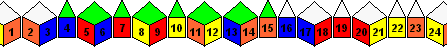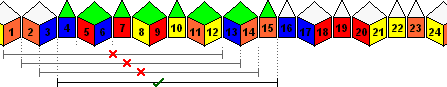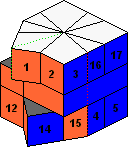# Square 1, Proof of counting formula

On the main Square-1 page a formula was mentioned for calculating the number of positions on the Square-1. In its most generic form it's 8(C+E-1)! / (2C+E), where there are C corner pieces and E edge pieces (and E>0). For the standard puzzle C=E=8 and this gives 15!/3 positions. On this page I will try to explain Mike Godfrey's remarkable proof of this.

When counting the number of positions, the problem is that corner pieces take twice a much room as the edge pieces. You cannot count it like most other puzzles, where you imagine assembling the puzzle piece by piece, and simply multiply together how much choice you have at each step. Since you must fill the two layers exactly, it seems you always have to keep track of how many corners are in each layer, just like we did on the main page. If there were only one big layer to fill instead of two, then it would be much easier.

The crux of Mike's proof is that we can do just that - put the pieces in one big circular layer - because those positions correspond exactly to arrangements of the pieces in the two layers of the Square-1.

Suppose all the pieces were somehow placed in a single large layer. I have illustrated this below as a single row, where the ends wrap around. How many ways are there of filling this with the 16 pieces?Lets place the white-orange edge first. There are 24 places in the circle where it can be. Once that has been placed, the other 15 pieces can be placed one by one in any order to the right of that. This means there are 24·15! possible arrangements as a single layer.

Assume for a moment that the previously mentioned correspondence holds, and that there are therefore 24·15! ways of placing the pieces in the two layers of the Square-1. To get the number of positions where rotations of the up/down layers are not considered different, we must divide by a factor of 12 for each layer, giving 15!/6 positions. The middle layer gives an extra factor of 2 to bring the final total to 15!/3. All that remains now is to show why that correspondence holds.

Start with an arrangement of the pieces in one large circle. To split it over two layers, we need to find a block of adjacent pieces that fills exactly 12 places, so that it can cover one of the layers. In the picture below, the first block of 12 that does that is 4-15. You can see that the sliding window of length 12 is blocked at any previous spot by a corner at one end or the other.The sliding window must at some point find a block of pieces that fits. The only way no such block could be found would be if we always have a corner alternately at one end or the other of the window. This of course cannot happen because there are edge pieces in the circle.

Now we can start transferring the pieces from the circle to the top layer of the puzzle, from left to right, starting from the front seam. When we get to piece 4, the start of the block of 12, it goes on the bottom layer directly below where you would have placed it otherwise. The rest of the pieces in that block also go upside down on the bottom, each one to the right of the last. When those have been placed, continue on the top layer with the remaining pieces.Conversely, it is quite easy to go the other way too. Given some position of the Square-1, we can extract the unique single layer arrangement that generates it. Simply go around the top layer, removing the pieces one by one and placing the in the large circle. At the first opportunity where the seams in the top and bottom layer match, switch to the bottom layer. After the bottom layer pieces have all been removed, do the remainder of the top layer.

There will always be some point where the top and bottom layer have a matching seam. The only way no matching seam could be found would be if we have just corners in both layers, with the layers out of sync. This of course cannot happen because there are edge pieces in the puzzle.

It is important that these two procedures are exact inverses of each other. Note especially how the sliding window we used on the circle corresponds to the presence or not of a matching seam in the square-1 layers. This shows each Square-1 position belongs to a unique circle arrangement, and therefore there are as many of one as there are of the other.

With this it also becomes easy to count the positions of a generalised version of the puzzle, for example one with C corners and E edges. These pieces cover 2C+E locations, so there are 2C+E ways to place the first edge piece in the circle. The remaining C+E-1 pieces have (C+E-1)! arrangements, giving a total of (2C+E)·(C+E-1)! arrangements. Each layer of the puzzle has (2C+E)/2 possible rotations, and dividing that out twice gives 4(C+E-1)!/(2C+E) positions of the general puzzle. If you don't ignore the middle layer, you get 8(C+E-1)!/(2C+E) positions.

We could bandage pieces together to create not only edges and corners, but also pieces that take up 3 or more times as much space as a single edge. When we do that however, the techniques above usually fail. The sliding window is then not guaranteed to be able to find a block of pieces in the circle arrangement that can serve as the bottom layer. Conversely, there is no guarantee that there is always some place on the puzzle where the two layers have a matching seam.

Back to main Square-1 page